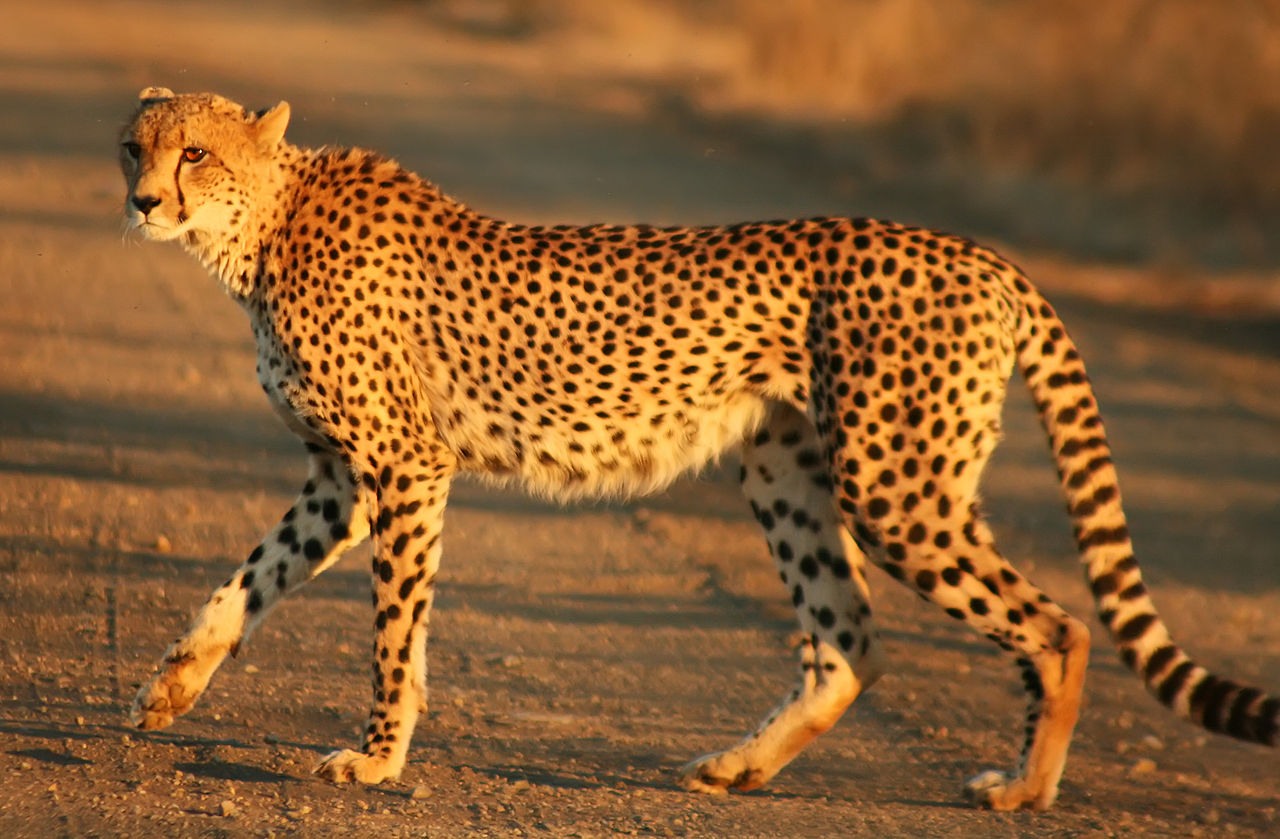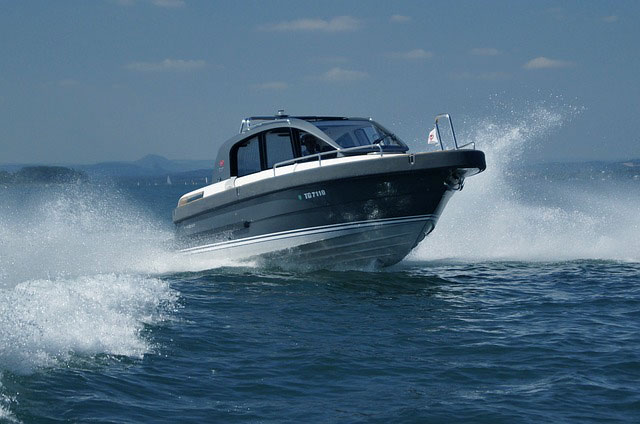10 minutes maximum! Can you do it in 5?

1. Which of these is the correct formula for speed?

• A. speed = distance x time
• B. speed = distance x acceleration
• C. speed = distance ÷ time
• D. speed = distance ÷ acceleration

2. A tortoise is pretty slow, only covering 100 cm in 50 seconds. It therefore has a speed of:

• A. 2 m/s
• B. 2 cm/s
• C. 0.5 m/s
• D. 0.5 cm/s

3+4. A cheetah can run at a top speed of 30 m/s.3. How far could it run in 5 seconds?
• A. 0.16 m
• B. 6 m
• C. 150 m
• D. 15 m

4. How long would it take the cheetah to cover 600 m?

• A. 20 s
• B. 0.05 s
• C. 200 s
• D. 18000 s

5. Which of these is the correct formula for acceleration?

• A. acceleration = velocity x time
• B. acceleration = change in velocity x time
• C. acceleration = velocity ÷ time
• D. acceleration = change in velocity ÷ time

6. What are the correct units for acceleration?

• A. "meters per second"
• B. "meters seconds"
• C. "meters seconds squared"
• D. "meters per second squared"
7-9. What are the missing numbers in this table showing the acceleration of different motor boats?acceleration (m/s2) starting velocity (m/s) final velocity (m/s) Time (s) 7. ? 0 0.25 4 100 0 20 5 8. 3 0 30 ? 0.1 1 10 90 9. 4 5 ? 20 2 40 45 10

10. A toy car accelerates across the floor at 2 m/s2. After 3 seconds the velocity is 10 m/s. What was the starting velocity?

• A. 60 m/s
• B. 10 m/s
• C. 6 m/s
• D. 4 m/s